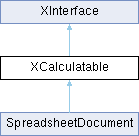LibreOffice LibreOffice 7.4 SDK API Reference
XCalculatable Interface Referencepublished

represents something that can recalculate. More...

`import"XCalculatable.idl";`

Inheritance diagram for XCalculatable:## Public Member Functions

void calculate ()
recalculates all dirty cells. More...

void calculateAll ()
recalculates all cells. More...

boolean isAutomaticCalculationEnabled ()
returns whether automatic calculation is enabled. More...

void enableAutomaticCalculation ([in] boolean bEnabled)
enables automatic calculation. More...Public Member Functions inherited from XInterface
any queryInterface ([in] type aType)
queries for a new interface to an existing UNO object. More...

void acquire ()
increases the reference counter by one. More...

void release ()
decreases the reference counter by one. More...

## Detailed Description

represents something that can recalculate.

## ◆ calculate()

 void calculate ( )

recalculates all dirty cells.

This calculates all formula cells which have not yet been calculated after their precedents have changed.

## ◆ calculateAll()

 void calculateAll ( )

recalculates all cells.

## ◆ enableAutomaticCalculation()

 void enableAutomaticCalculation ( [in] boolean bEnabled )

enables automatic calculation.

With automatic calculation, each formula cell is recalculated whenever its value is needed after its precedents have changed. The value is needed if the cell is displayed or used in another calculation.

Parameters
 bEnabled `TRUE` to enable automatic calculation, `FALSE` to disable.

## ◆ isAutomaticCalculationEnabled()

 boolean isAutomaticCalculationEnabled ( )

returns whether automatic calculation is enabled.

With automatic calculation, each formula cell is recalculated whenever its value is needed after its precedents have changed. The value is needed if the cell is displayed or used in another calculation.

Returns
`TRUE`, if automatic calculation is enabled.

The documentation for this interface was generated from the following file: